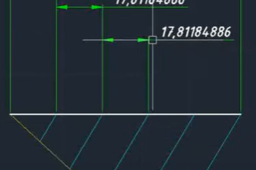# How to divide undefined lineI will show you how to divide the line by 5 pieces in link video.

1. ### Step 1: draw a line at an angle less than 90 degrees to the line to be divided

Draw a line at an angle less than 90 degrees to the line to be divided

See the following video tutorial

2. ### Step 2: Divide the lines that have been made in step 1 as needed

Divide the lines that have been made in step 1 as needed.

See the following video tutorial

3. ### Step 3: Draw a line that connects the ends of the line that has been divided and that has been divided.

Draw a line that connects the ends of the line that has been divided and that has been divided.

See the following video tutorial

4. ### Step 4: Draw a line parallel to the line in step 3 that is at each end of the divided line.

Draw a line parallel to the line in step 3 that is at each end of the divided line.

See the following video tutorial